## A Month of Math Software – February 2011

February 28th, 2011

Last month I started a new feature called a Month of Math Software and since it turned out to be quite popular I thought I’d do it again. If you have any math software news or reviews that you’d like included in next month’s edition then feel free to contact me.

Releases – Commercial
Magma V2.17-5 has been released and the list of changes is here. Magma is a large software package designed for computations in algebra, number theory, algebraic geometry and algebraic combinatorics.

Releases – Open Source
A major new version of Octave, a high quality, open-source alternative to MATLAB, has been released. Version 3.4.0 has been available from February 8th and the changelog is available at www.gnu.org/software/octave/NEWS-3.4.html

A new mathematics package for Mac OS X has been produced – EureKalc 3.  Screenshot below.  Currently at version 3.ß.02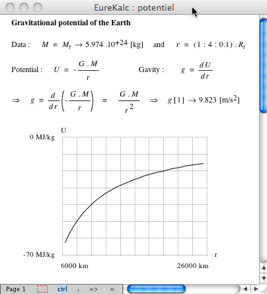Version 11 beta of Euler Math Toolbox has been released with some new symbolic functionality.  There is a blog post on the new functionality at http://sourceforge.net/apps/wordpress/eumat/2011/02/07/version-11/

A new stable release of Gnumeric (The spreadsheet component of GNOME Office) has been released.  The new version is 1.10.3 and recent changes can be found at http://projects.gnome.org/gnumeric/announcements/1.10/gnumeric-1.10.13.shtml

Optimization
Professor Stan Uryasev from the University of Florida has produced a website containing a suite of optimization test problems.

Version 2 of Sergey Moiseev’s free DirectSearch optimization package for Maple has been released. The DirectSearch package is a collection of commands to numerically compute local and global minimums (maximums) of nonlinear multivariate function with (without) constraints.

The Numerical Algorithms Group (NAG) discusses the double-edged sword of SSE instructions in Wandering Precision.  SSE instructions can make your numerical algorithms significantly faster but this comes at a price.

Non Deterministic Floating Point conversions in Java.  More SSE weirdness; a very detailed article that explains an odd bug in Java on certain architectures.

## Mathematica – The difference between Equal and SameQ

February 22nd, 2011

A Mathematica user contacted me recently and asked why

(1/16 Cos[a] (Cos[b] + 15 Cos[3 b])) === (1/8 Cos[b] (-7 + 15 Cos[2b])Cos[a])


returned False when he expected True. Let’s take a look at what is going on here.

The SameQ command (===) and the Equal command (==) are subtly different.  Equal (==) tests to see if two expressions are mathematically equivalent whereas SameQ tests to see if they are structurally equivalent.  In general, SameQ is the stricter of the two tests and is often too strict for our needs.

A very simple example

The simplest example might be to compare 2 and 2. (That is 2 with a period at the end)

Mathematically these are the same since they both represent the number two. However, from a Mathematica structural point of view, they are different since one has infinite precision whereas the other has double precision. So, we have

2==2. gives True
2===2. gives False

A more complicated example

Sqrt + Sqrt == Sqrt[5 + 2 Sqrt] which gives True
Sqrt + Sqrt === Sqrt[5 + 2 Sqrt] which gives False


Mathematically the above is always true but the structure of the expressions on the LHS and RHS are different under normal evaluation rules.

So, what do I mean by ‘structurally equivalent?’.  To be honest, I am slightly woolly on this myself but in my head I consider two expressions to be structurally equivalent if their TreeForms are the same

TreeForm[Sqrt + Sqrt ]TreeForm[Sqrt[5 + 2 Sqrt] ]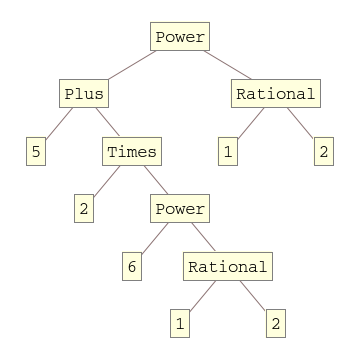The important thing to remember here is that the argument to TreeForm is evaluated by Mathematica before it gets passed to TreeForm. So, the above images show us that under normal evaluation rules, these two expressions have different structural forms.  In the next example we’ll see how this detail can matter

Exp[I Pi] and all that

Let’s look at

Exp[I Pi] === -1


Would that be True or False based on what we have seen so far?  It is certainly mathematically true but remember that a triple equals requires both sides to be structurally equivalent and at first sight it appears that this isn’t the case here. You might expect Exp[I Pi] to have a very different TreeForm from -1 and so you’d expect the above to evaluate to False. However if you do

TreeForm[Exp[I Pi]]


then you get a single box containing -1. This occurs because Mathematica evaluates Exp[I Pi] to -1 before passing to TreeForm. This evaluation also occurs when you do Exp[I Pi] === -1 so what you are really testing is -1===-1 which is obviously True.

Back to the original example

(1/16 Cos[a] (Cos[b] + 15 Cos[3 b])) === (1/8 Cos[b] (-7 + 15 Cos[2b])Cos[a])


If you use === then it returns False and this is ‘obviously’ the case because if you evaluate the TreeForms of both expressions then you’ll see that they get stored with different structural forms after being evaluated.

TreeForm[(1/16 Cos[a] (Cos[b] + 15 Cos[3 b]))]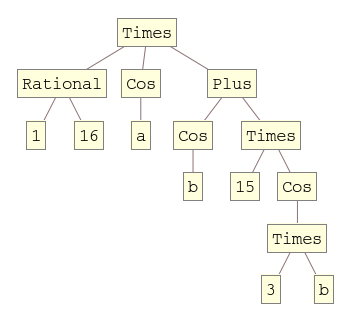TreeForm[(1/8 Cos[b] (-7 + 15 Cos[2 b]) Cos[a])]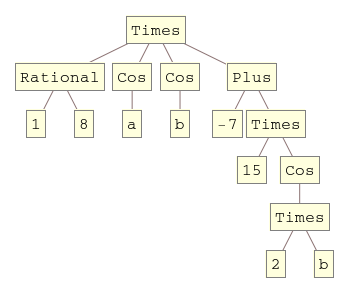Since we are interested in mathematical equivalence, however, we use ==

(1/16 Cos[a] (Cos[b] + 15 Cos[3 b])) == (1/8 Cos[b] (-7 + 15 Cos[2 b]) Cos[a])


What happens this time, however, is that Mathematica returns the original expression unevaluated. This is because, under the standard set of transformations that Mathematica applies when it evaluates ==, it can’t be sure one way or the other and so it gives up.

To proceed we wrap the whole thing in FullSimplify which essentially says to Mathematica ‘Use everything you’ve got to try and figure this out – no matter how long it takes’  I.e. you allow it to use more transforms.

FullSimplify[(1/16 Cos[a] (Cos[b] + 15 Cos[3 b])) == (1/8 Cos[b] (-7 + 15 Cos[2 b]) Cos[a])]

True


Finally, we have the result that the user expected.

## Carnival of Mathematics #74 – The Tungsten Edition

February 8th, 2011

Welcome to the slightly delayed Carnival of Mathematics #74 – Tungsten Edition.  In a departure from COM tradition, this edition is on the same blog as the last edition due to a lack of hosting volunteers.  Fortunately, however, the volunteers are starting to turn up and so this is the last month that you’ll have to put up with me for a while.  Next month will see the carnival hosted by Daniel over at General Musings and April will be taken care of by AcmeScience (thanks peeps!).  The rest of the year is up for grabs so if you’d like to host a future carnival then contact me and we’ll work something out.

One tradition that I’m not about to give up on, however, is the number trivia section.  So, let’s see what 74 has for us.  In stark contrast to 73, the Chuck Norris of Numbers, 74 doesn’t seem to have much going for it.  It’s probably overweight for a start since it is the 3rd hungry number (hungry because it tries to eat as much Pi as possible).  Other than that it is odious, semiprime and altogether rather dull!

Enough of the trivia and on with the show.

First up we have a post from Datavisualization.ch which shows a set of infographics from around 150 years ago.  Much of this work is rather beautiful and demonstrates that the field of infographics is much older than you might think.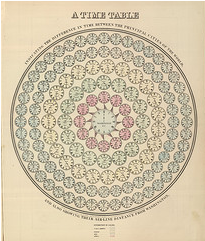For a rather more modern take on statistics and infographics I highly recommend the UK BBC Four TV documentary, The Joy of Stats.  This was a one off show broadcast in late 2010 and hosted by Hans Rosling (Also the star of a brilliant TED talk).  Several clips of the show are available over at The Open University and it was recently mentioned by The Royal Statistical Society.

From statistics and onto the theory of numbers with a post by Matt Springer called ‘Sunday Function’.  In this post, Matt demonstrates a wonderfully simple proof concerning fractions which have terminating decimal expansions.  It turns out that it just happened to be a Sunday morning when I read this delightful little post over coffee and it set me up for the day.

Number theory has been big news in the mathematical world recently thanks to some work by Ken Ono and colleagues who have found a finite, algebraic formula for partition numbers.  The original papers can be found at http://www.aimath.org/news/partition/ (thanks to everyone on twitter who sent me that) and there has been discussion all over the web including at Wired.com and The Language of Bad Physics.  Emory University (where Ken works) have a press-release but the best resource I’ve found so far is an informal talk by the man himself at YouTube (see below).

One of my biggest personal interests is mathematical software and so my contribution to this month’s carnival is a round-up of mathematical software news for January.  January also saw a post from Wolfram Research on how to use some of Mathematica’s new control theory functions to stabilize an inverted pendulum which also serves as a nice introduction to some standard control theory techniques.

Another mathematical software article that was brought to my attention last month was a post from the Mathcad team concerning a very simple looking integral.  Computers often do symbolic calculus using different techniques to human beings and this is occasionally reflected in the results.  Check out the article for the details.

On a lighter note, we have Music is Math: Ten Songs about Mathematics from Dave Richeson of ‘Division by Zero’ fame.  My favourite of the bunch is ‘A finite simple Group of Order 2’ (below) which I’ve some across before.  The rest of them, however, are new to me and a lot of fun (and in a couple of cases, quite nice to listen to).

My boss used to be a research mathematician and is something of a pedant (In a good way Chris…in a good way).  It seems that this is a trait he has in common with Peter Rowlett, author of Travels in a mathematical world, who has written a piece called Pedantry on Euler and Masts where ﻿﻿he investigates the facts behind an old Euler story.  There’s also some interesting discussion of the difficulty of presenting maths for the masses.

Whereas I do Random Walking, Sander Huisman does Random Hopping and in the process he comes up with some great looking pictures and interesting mathematics.John D. Cook compares the iPhone to the method of least squares in When it works, it works really well, Ed4All shows us a mental arithmetic short cut for squares ending in five and Math-Frolic presents A Seemingly Impossible Task, That Isn’t.

Last but by no means least, Guillermo Bautista, organiser of the Math and Multimedia blog carnival, gives us an introduction to similarity while Alasdair’s Musings brings us A cute result relating to sums of cubes.